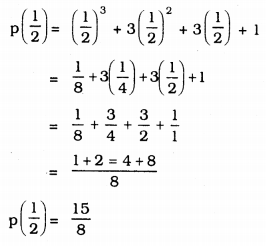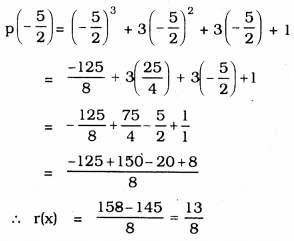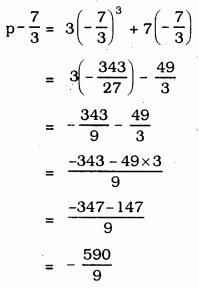# KSEEB Solutions for Class 9 Maths Chapter 4 Polynomials Ex 4.3

In this chapter, we provide KSEEB SSLC Class 9 Maths Chapter 4 Polynomials Ex 4.3 for English medium students, Which will very helpful for every student in their exams. Students can download the latest KSEEB SSLC Class 9 Maths Chapter 4 Polynomials Ex 4.3 pdf, free KSEEB SSLC Class 9 Maths Chapter 4 Polynomials Ex 4.3 pdf download. Now you will get step by step solution to each question.

## Karnataka Board Class 9 Maths Chapter 4 Polynomials Ex 4.3

Question 1.
Find the remainder when x3 + 3x2 + 3x + 1 is divided by
i) x + 1
ii) x−12
iii) x
iv) x + π
v) 5 + 2x
i) p(x) = x3 + 3x2 + 3x + 1 g(x) = x – 1
Let x – 1 = 0, then
x = 1.
As per Remainder theorem, r(x) = p(x) = p(a)
p(x) = x3 + 3x2 + 3x + 1
p(1) = (1)3 + 3(1)2 + 3(1) + 1
= 1 + 3(1) + 3(1) + 1
= 1 + 3 + 3 + 1
P(1) = 8
∴ r(x) = p(x) = 8
∴ Remainder is 8.

ii) p(x) = x3 + 3x2 + 3x + 1 g(x) = x−12
If x−12=0 then x=12
p(x) = x3 + 3x2 + 3x + 1∴ r(x) = p(x) = p(a) = 158
∴ Remainder is 158

iii) p(x) = x3 + 3x2 + 3x + 1
g(x) = x
If x = 0, then
p(x) = x3 + 3x2 + 3x + 1
p(0) = (0)3 + 3(0)2 + 3(0) + 1
= 0 + 3(0) + 3(0) + 1
= 0 + 0 + 0 + 1
p(0) = 0
Remainder r(x) = 1.

iv) p(x) = x3 + 3x2 + 3x + 1
g(x) = x + π
If x + π = 0, then x = -π
p(x) = x3 + 3x2 + 3x + 1
p(-π) = (-π)3 + 3(-π)2 + 3(-π) + 1
p(-πt) = -π23 – 3π2 – 3π + 1
r(x) = -π3 – 3v2 – 3π+1

v) p(x) = x3 + 3x2 + 3x + 1
g(x) = 5 + 2x
If 5 + 2x = 0, then 2x = -5
x=−52
p(x) = x3 + 3x2 + 3x + 1Question 2.
Find the remainder when x3 – ax2 + 6x – a is divided by x – a.
p(x) = x3 – ax2 + 6x – a
If g(x) = x – a, then r(x) = ?
Let x – a = 0, then x = a
p(x) = x3 – ax2 + 6x – a
∴ p(a) = (a)3 – a(a)2 + 6(a) – a
= a3 – a3 + 6a – a
∴ p(a) = 5a
∴ r(x) = p(a) = 5a.

Question 3.
Check whether 7 + 3x is a factor of 3x3 + 7x.
p(x) = 3x3 + 7x
Let g(x) = 7 + 3x = 0. then
If 7 + 3x = 0, then 3x = -7
x=−73
If p(x) is divided by p(z), remainder r(x) – 0, then g(x) is a factor.
p(x) = 3x3 + 7xHere, r(x)=−5909. This is not equal to Zero.
Hence 7 + 3x is not a factor of p(x).

All Chapter KSEEB Solutions For Class 9 Maths

—————————————————————————–

All Subject KSEEB Solutions For Class 9

*************************************************

I think you got complete solutions for this chapter. If You have any queries regarding this chapter, please comment on the below section our subject teacher will answer you. We tried our best to give complete solutions so you got good marks in your exam.

If these solutions have helped you, you can also share kseebsolutionsfor.com to your friends.

Best of Luck!!